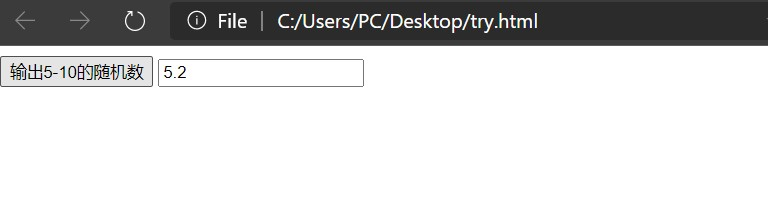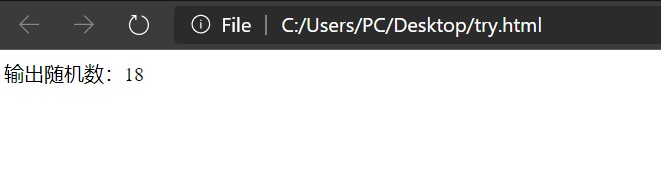# html生成随机数（小数或整数）并以按钮形式输出

## 代码

### 按钮形式

<script>
function Num()
{
document.getElementById("field").value=Math.floor(Math.random()*5+5) + Math.ceil(Math.random()*10)/10
}
</script>
<button onclick="Num()">输出5-10的随机数</button> <input type="text" id="field">


### 文本形式

<script language="javascript">
var a=parseInt(Math.random()*100);
document.write("输出随机数：",a);
</script>


### 效果按钮形式（可多次按下） 文本形式

## 语法

 Math.ceil() 向上取整。 Math.floor() 向下取整。 Math.round() 四舍五入。 Math.random() [0.0 ~ 1.0) 区间一个伪随机数

 Math.ceil(Math.random()*10) 获取从1到10的随机整数 ，取0的概率极小。 Math.round(Math.random()) 可均衡获取0到1的随机整数。 Math.floor(Math.random()*10) 可均衡获取0到9的随机整数。 Math.round(Math.random()*10) 获取0到10的随机整数，其中获取最小值0和最大值10的几率少一半。

## 参考

05-29133506-261448
09-133292
12-294545
03-26965
08-26366
08-308863
09-062071
07-08310
12-152336